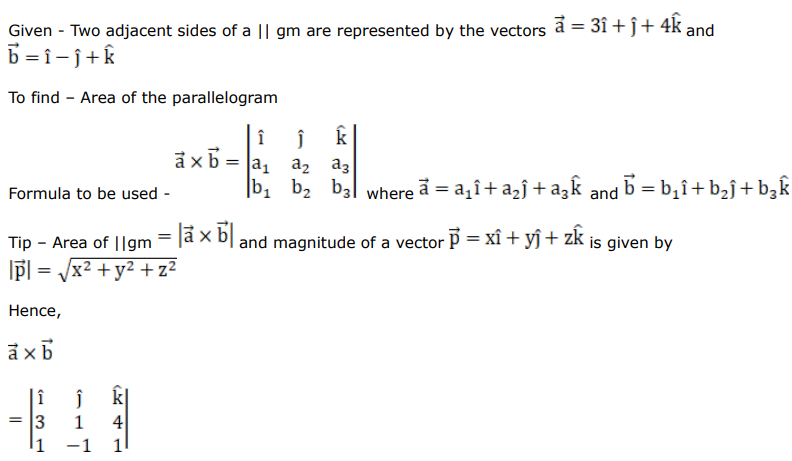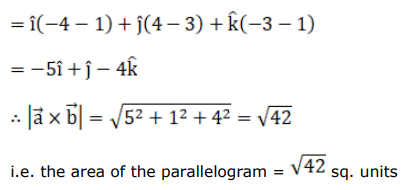# Mark against the correct answer in each of the following:

Question:

Mark $(\sqrt{)}$ against the correct answer in each of the following:

Two adjacent sides of a $\| \mathrm{gm}$ are represented by the vectors $\vec{a}=(3 \hat{i}+\hat{j}+4 \hat{k})$ and $\overrightarrow{\mathrm{b}}=(\hat{\mathrm{i}}-\hat{\mathrm{j}}+\hat{\mathrm{k}})$ The area of the II gm is

A. $\sqrt{42}$ sq units

B. 6 sq units

C. $\sqrt{35}$ sq units

D. none of these

Solution: